# NCERT Solutions for Class 12 Maths Chapter 6 Application of Derivatives Ex 6.2

These NCERT Solutions for Class 12 Maths Chapter 6 Application of Derivatives Ex 6.2 Questions and Answers are prepared by our highly skilled subject experts.

## NCERT Solutions for Class 12 Maths Chapter 6 Application of Derivatives Exercise 6.2Question 1.
Show that the function given by f(x) = 3x + 17 is strictly increasing on R.
Solution:
f(x) = 3x + 17
Since f(x) is a polynomial function, it is continuous and differentiable in R
∴ f'(x) = 3 > 0 for all x ∈ R
Hence f'(x) is strictly increasing on R

Question 2.
Show that the function given by f (x) = e2x is strictly increasing on R.
Solution:
f (x) = e2x
Since f(x) is an exponential function, it is continuous and differentiable in R
∴ f (x) = e2x > 0 for all x ∈ R
Hence f(x) is strictly increasing on R.

Question 3.
Show that the function given by f (x) = sin x is
(a) strictly increasing in $$\left( 0,\frac { \pi }{ 2 } \right)$$
(b) strictly decreasing in $$\left( \frac { \pi }{ 2 } ,\pi \right)$$
(c) neither increasing nor decreasing in (0, π)
Solution:
We have f(x) = sinx
∴ f’ (x) = cosx
(a) f’ (x) = cos x is + ve in the interval $$\left( 0,\frac { \pi }{ 2 } \right)$$
⇒ f(x) is strictly increasing on $$\left( 0,\frac { \pi }{ 2 } \right)$$

(b) f’ (x) = cos x is a -ve in the interval $$\left( \frac { \pi }{ 2 } ,\pi \right)$$
⇒ f (x) is strictly decreasing in $$\left( \frac { \pi }{ 2 } ,\pi \right)$$

(c) f’ (x) = cos x is +ve in the interval $$\left( 0,\frac { \pi }{ 2 } \right)$$
while f’ (x) is -ve in the interval $$\left( \frac { \pi }{ 2 } ,\pi \right)$$
∴ f(x) is neither increasing nor decreasing in (0, π)Question 4.
Find the intervals in which the function f given by f(x) = 2x² – 3x is
(a) strictly increasing
(b) strictly decreasing
Solution:
f(x) = 2x² – 3x
⇒ f’ (x) = 4x – 3
⇒ f’ (x) = 0 at x = $$\frac { 3 }{ 4 }$$
The point $$x=\frac { 3 }{ 4 }$$ divides the real(i) f(x) is strictly increasing in ($$\frac { 3 }{ 4 }$$, ∞)
(ii) f(x) is strictly increasing in (- ∞, $$\frac { 3 }{ 4 }$$)

Question 5.
Find the intervals in which the function f given by f (x) = 2x³ – 3x² – 36x + 7 is
(a) strictly increasing
(b) strictly decreasing
Solution:
f (x) = 2x³ – 3x² – 36x + 7
Differentiating w.r.t. x,
f (x) = 6 (x – 3) (x + 2)
⇒ f’ (x) = 0 at x = 3 and x = – 2
The points x = 3, x = – 2, divide the real line into three disjoint intervals viz. (-∞, -2), (-2, 3), (3, ∞)
Now f’ (x) is +ve in the intervals (-∞, -2) and (3, ∞). Since in the interval (-∞, -2) each factor x – 3, x + 2 is -ve.
⇒ f’ (x) = + ve.
(a) f is strictly increasing in (-∞, -2)∪(3, ∞)

(b) In the interval (-2, 3), x+2 is +ve and x-3 is -ve.
f (x) = 6(x – 3)(x + 2) = + x – = -ve
∴ f is strictly decreasing in the interval (-2, 3).Question 6.
Find the intervals in which the following functions are strictly increasing or decreasing:
(a) x² + 2x – 5
(b) 10 – 6x – 2x²
(c) – 2x3 – 9x² – 12x + 1
(d) 6 – 9x – x²
(e) (x + 1)3(x – 3)3
Solution:
(a) Let f(x) = x² + 2x – 5
Differentiating w.r.t. x,
f'(x) = 2x + 2 = 2(x + 1)
f'(x) = 0 ⇒ 2(x + 1) = 0 = – 1
x = – 1 divides R into disjoint open intervals as (-∞, -1) and (-1, ∞)∴ f is strictly decreasing in (-∞, -1)
f is strictly increasing in (-1, ∞)

(b) Let f(x) = 10 – 6x – 2x²
Differentiating w.r.t. x,
f'(x) = – 6 – 4x = – 2(2x + 3)
f'(x) = 0 ⇒ – 2(2x + 3) = 0∴ f is strictly decreasing in (-∞, $$\frac { -3 }{ 2 }$$) and
f is strictly increasing in ($$\frac { 3 }{ 2 }$$, ∞)

(c) Let f(x) = – 2x3 – 9x2 – 12x + 1
∴ f’ (x) = – 6x2 – 18x – 12
= – 6(x2 + 3x + 2)
f'(x) = – 6(x + 1)(x + 2), f’ (x) = 0 gives x = -1 or x = -2
The points x = – 2 and x = – 1 divide the real line into three disjoint intervals namely ( – ∞, – 2) ( – 2, – 1) and( – 1, ∞).f (x) is increasing in (-2, -1)
In the interval (-1, ∞) i.e.,-1 < x < ∞,(x + 1) and (x + 2) are both positive. f’ (x) = (-) (+) (+) = -ve.
⇒ f (x) is decreasing in (-1, ∞)
Hence, f (x) is increasing for – 2 < x < – 1 and decreasing for x < – 2 and x > – 1.

(d) Let f(x) = 6 – 9x – x²
Differentiating w.r.t. x,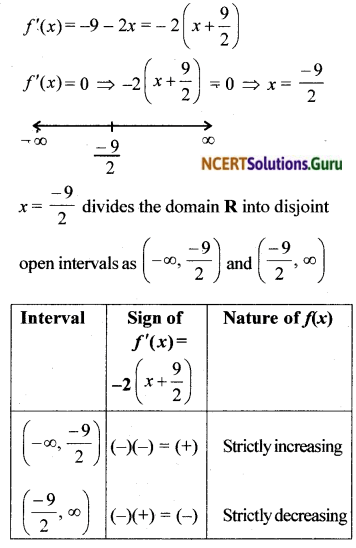(e) Let f(x) = (x + 1)3(x – 3)3
Differentiating w.r.t. x,
f'(x) = 3 (x² – 2x – 3)² (2x – 2)
= 6 [(x + 1) (x – 3)]² (x – 1)
= 6 (x + 1)² (x – 1 ) (x – 3)²
f'(x) = 0 ⇒ x = – 1, 1 or 3
x = – 1, x = 1 or x = 3 divide R into disjoint open intervals (-∞, -1), (-1, 1), (1, 3) and (-3, – ∞)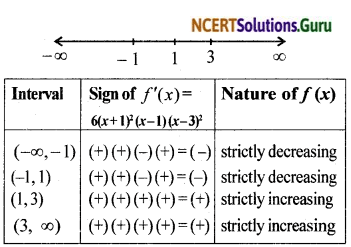Thus f is strictly decreasing in (- ∞, – 1) and (- 1, 1) and f is strictly increasing in (1, 3) and (3, ∞)Question 7.
Show that y = log(1 + x) – $$\frac { 2x }{ 2 + x }$$, x > – 1 is an increasing function of x throughout its domain.
Solution:When x > – 1, we get y’ = (+)(+) = (+) i.e.,
y’ is positive for x > – 1
∴ When x > – 1, y = log(1 + x) – $$\frac { 2x }{ 2 + x }$$ an increasing function.

Question 8.
Find the values of x for which y = [x (x – 2)]² is an increasing function.
Solution:
y = x4 – 4x3 + 4x2
∴ $$\frac { dy }{ dx }$$ = 4x3 – 12x2 + 8x
For the function to be increasing $$\frac { dy }{ dx }$$ >0
4x3 – 12x2 + 8x>0
⇒ 4x(x – 1)(x – 2)>0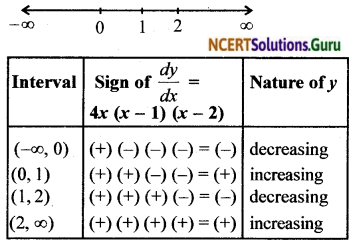Thus, the function is increasing for 0 < x < 1 and x > 2.

Question 9.
Prove that y = $$\frac { 4sin\theta }{ (2+cos\theta ) } -\theta$$ is an increasing function of θ in $$\left[ 0,\frac { \pi }{ 2 } \right]$$
Solution: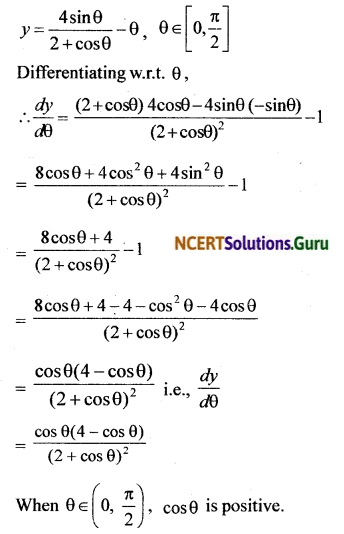∴ $$\frac { dy }{ dθ }$$ > 0 since (4 – cos θ) is positive.
Hence y is strictly increasing on (0, $$\frac { π }{ 2 }$$).
Also y is continuous at θ = 0 and θ = $$\frac { π }{ 2 }$$.
Hence y is increasing on [0, $$\frac { π }{ 2 }$$]Question 10.
Prove that the logarithmic function is strictly increasing on (0, ∞).
Solution:
Let f (x) = log x.
The domain of f is (0, ∞).
Now, f’ (x) = $$\frac { 1 }{ x }$$
When takes the values x > 0
$$\frac { 1 }{ x }$$ > 0, when x > 0,
∵ f’ (x) > 0
Hence, f (x) is an increasing function for x > 0 i.e

Another method:
From the graph, we can observe that as x increases f(x) also increases. Hence logx is an increasing function on (0, ∞).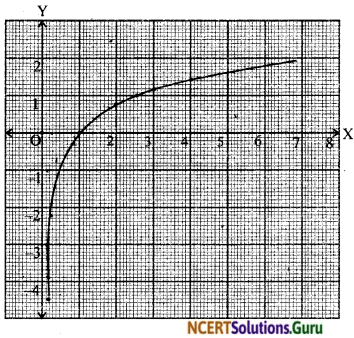Question 11.
Prove that the function f given by f (x) = x² – x + 1 is neither strictly increasing nor strictly decreasing on (- 1, 1).
Solution:
let f (x) = x² – x + 1
Differentiating both sides w.r.t. x,
f'(x) = 2x – 1
f'(x) = 2(x – $$\frac { 1 }{ 2 }$$) … (1)
f'(x) = 0 ⇒ 2(x – $$\frac { 1 }{ 2 }$$) = 0 ⇒ x = $$\frac { 1 }{ 2 }$$
∴ The domain of f(x) is (-1, 1)∴ f(x) is strictly increasing in ($$\frac { 1 }{ 2 }$$, 1) and strictly decreasing in (- 1, $$\frac { 1 }{ 2 }$$)
In the interval (- 1, 1) the given function is neither strictly increasing nor strictly decreasing.

Question 12.
Which of the following functions are strictly decreasing on $$\left[ 0,\frac { \pi }{ 2 } \right]$$
i. cos x
ii. cos 2x
iii. cos 3x
iv. tan x
Solution: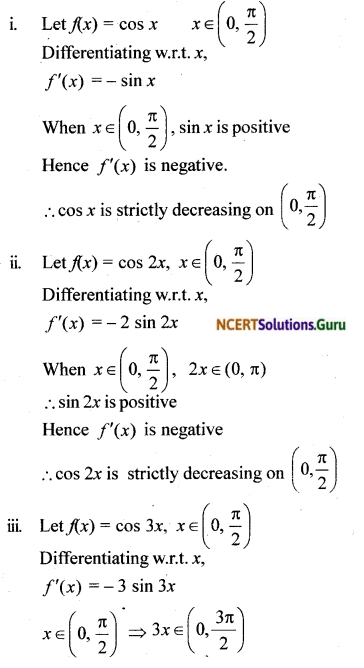sin 3x can be positive or negative.
∴ f'(x) can be positive or negative.
Hence cos 3x is neither increasing nor decreasing on (0, ($$\frac { π }{ 2 }$$).

iv. Let f(x) = tan x, x ∈ (0, $$\frac { π }{ 2 }$$)
Differentiating w.r.t. x,
f'(x) = sec²x is positive
tan x is strictly increasing on (0, $$\frac { π }{ 2 }$$)
Thus cos x and cos 2x are strictly decreasing on (0, $$\frac { π }{ 2 }$$)Question 13.
On which of the following intervals is the function f given by f (x) = x100 + sin x – 1 strictly decreasing ?
i. (0, 1)
ii. $$\left[ \frac { \pi }{ 2 } ,\pi \right]$$
iii. $$\left[ 0,\frac { \pi }{ 2 } \right]$$
iv. none of these
Solution:
f(x) = x100 + sin x – 1
Differentiating w.r.t x,
∴ f’ (x)= 100x99 + cos x

i. When x ∈ (0, 1), x99 > 0 and cosx > 0
∴ f'(x) is positive
i.e.,/(x) is strictly increasing on (0, 1)

ii. When x ∈ ($$\frac { π }{ 2 }$$, π), 100x99 > 100 and -1 < cosx < 0
f'(x)= 100x99 + cosx > 100 + a negative number greater than – 1.
f'(x) is positive
∴ Hence f(x) is strictly increasing on ($$\frac { π }{ 2 }$$, π)

iii. When x ∈ (0, $$\frac { π }{ 2 }$$), 100x99 > 0 and cosx > 0
Hence f'(x) is positive
f(x) is strictly increasing on (0, $$\frac { π }{ 2 }$$)
∴ f(x) is not strictly decreasing in any of the intervals (0, 1), ($$\frac { π }{ 2 }$$, π) and (0, $$\frac { π }{ 2 }$$).

Question 14.
Find the least value of a such that the function f given by f (x) = x² + ax + 1 is strictly increasing on (1, 2).
Solution:
We have f (x) = x² + ax + 1
∴ f’ (x) = 2x + a.
Since f (x) is an increasing function on (1, 2)
f’ (x) > 0 for all 1 < x < 2 Now, f” (x) = 2 for all x ∈ (1, 2) ⇒ f” (x) > 0 for all x ∈ (1, 2)
⇒ f’ (x) is an increasing function on (1, 2)
⇒ f’ (x) is the least value of f’ (x) on (1, 2)
But f’ (x)>0 ∀ x∈ (1, 2)
∴ f’ (1)>0 ⇒ 2 + a > 0 ⇒ a > – 2
∴ Thus, the least value of ‘a’ is – 2.

Question 15.
Let I be any interval disjoint from (- 1, 1). Prove that the function f given by f(x) = x + $$\frac { 1 }{ x }$$ is strictly increasing on I.
Solution:
f(x) = x + $$\frac { 1 }{ x }$$ and I = R – (-1, 1)
Differentiating w.r.t. x,
f'(x) = 1 – $$\frac{1}{x^{2}}$$ = $$\frac{x^{2}-1}{x^{2}}$$
When x² > 1, f'(x) > 0
When x ∈ R – (- 1, 1), x² > 1
Hence f'(x) is positive.
i.e., when x ∈ 1, f(x) is strictly increasingQuestion 16.
Prove that the function f given by f (x) = log sin x is strictly increasing on (0, $$\frac { π }{ 2 }$$) and strictly decreasing on ($$\frac { π }{ 2 }$$, π)
Solution:
f'(x) = $$\frac { 1 }{ sin\quad x }$$cos x cot x
when 0 < x < $$\frac { \pi }{ 2 }$$, f’ (x) is +ve; i.e., increasing
When $$\frac { \pi }{ 2 }$$ < x < π, f’ (x) is – ve; i.e., decreasing,
∴ f (x) is decreasing. Hence, f is increasing on (0, π/2) and strictly decreasing on (π/2, π).

Question 17.
Prove that the function f given by f(x) = log cos x is strictly decreasing on (0, $$\frac { π }{ 2 }$$)and strictly increasing on ($$\frac { π }{ 2 }$$, π)
Solution:
Given f(x) = log cos x
f'(x) = $$\frac { 1 }{ cosx }$$ (-sinx) = – tanx
In the interval $$\left( 0,\frac { \pi }{ 2 } \right)$$, f’ (x) = -ve
∴ f is strictly decreasing.
In the interval $$\left( \frac { \pi }{ 2 } ,\pi \right)$$, f’ (x) is + ve.
∴ f is strictly increasing in the interval.

Question 18.
Prove that the function given by
f (x) = x3 – 3x2 + 3x -100 is increasing in R.
Solution:
f’ (x) = 3x2 – 6x + 3
= 3 (x2 – 2x + 1)
= 3 (x -1 )2
Now x ∈ R, f'(x) = (x – 1)2 ≥ 0
i.e. f'(x) ≥ 0 ∀ x ∈ R; hence, f(x) is increasing on R.Question 19.
The interval in which y = x2 e-x is increasing is
(a) (-∞, ∞)
(b) (-2 0)
(c) (2, ∞)
(d) (0, 2)
Solution:
y = x2 e-x
Differentiating w.r.t. x, we getx = 0 and x = 2 divide the domain R into disjoint open intervals as (-∞, 0), (0, 2) and (2, ∞).
∴ f is strictly increasing on (0, 2)

error: Content is protected !!